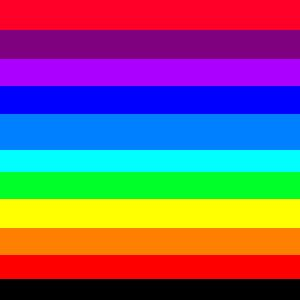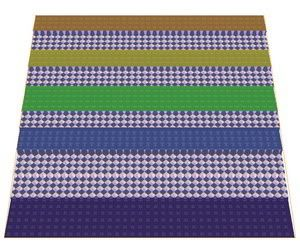en
CLOSURE ANNOUNCEMENT: The C&C forums will be closed on December 13th, 2023. Read more about the future of the forums here.

# RA3 WB 'open from TGA' colour setting

I don't know if anyone have already known about this or not, but i thought this is the right place to share it [:)]
I just found out that if you make targa (.tga) 32 bit colour with this setting:

height 350 (elevation 2), R=255, G=0, B=40 #ff0028
height 300, R=127, G=0, B=127 #7f007f
height 280 (elevation 1), R=171, G=0, B=255 #ab00ff
height 240, R=0, G=0, B=255 #0000ff
height 210 (elevation 0), R=0, G=127, B=255 #007fff
height 180, R=0, G=255, B=255 #00ffff
height 130 (shallow), R=0 G=255, B=40 #00ff28
height 60, R=255, G=255, B=0 #ffff00
height 30, R=255, G=127, B=0 #ff7f00
height 0, R=255, G=0, B=0 #ff0000
height 0 (deep), R=0, G=0, B=0 #000000then you will get this as a result:maybe this could help anyone who want to make shaped islands [:)]